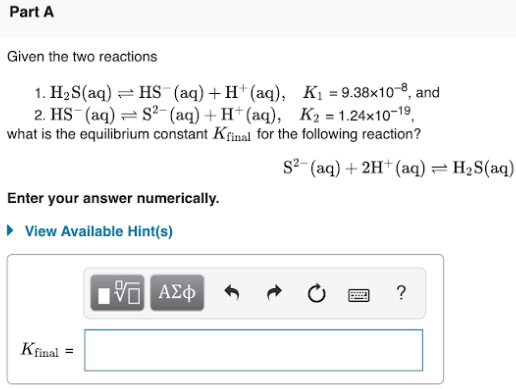# Given the two reactions: 1. H2S(aq) ⇌ HS^-(aq) + H^+(aq) K1 = 9.48 x 10^-8 and 2. HS^-(aq) ⇌ S^2-(aq) + H^+(aq) K2 = 1.24 x 10 -19 What is the equilibrium constant Kfinal for the following reaction? S^2-(aq) + 2H^+(aq) ⇌ H2S(aq) Enter your answer numerically.